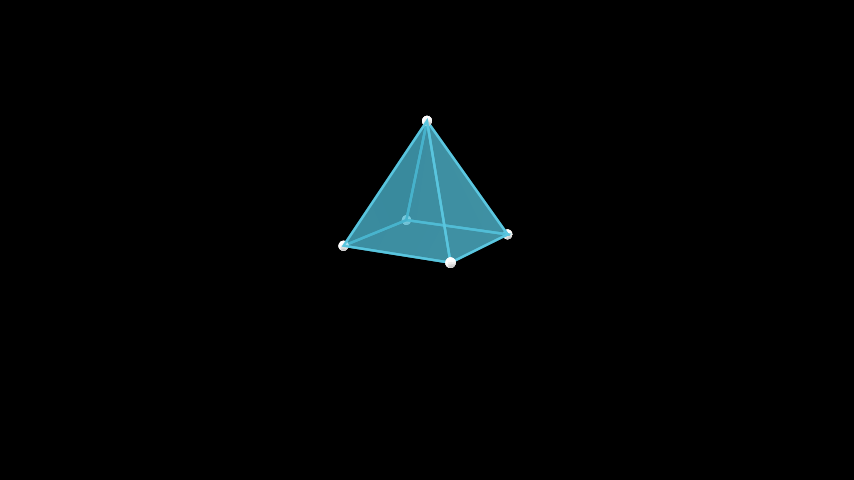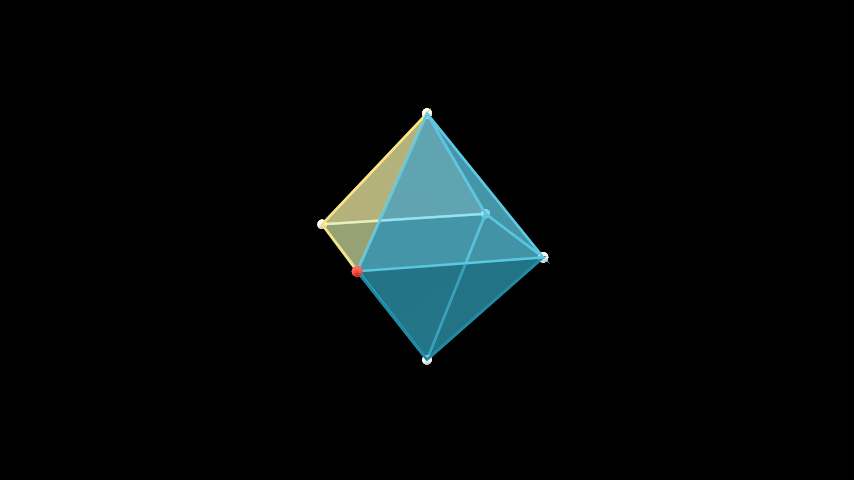# Polyhedron¶

Qualified name: `manim.mobject.polyhedra.Polyhedron`

class Polyhedron(vertex_coords, faces_list, faces_config={}, graph_config={})[source]

An abstract polyhedra class.

In this implementation, polyhedra are defined with a list of vertex coordinates in space, and a list of faces. This implementation mirrors that of a standard polyhedral data format (OFF, object file format).

Parameters
• vertex_coords (List[Union[List[float], numpy.ndarray]]) – A list of coordinates of the corresponding vertices in the polyhedron. Each coordinate will correspond to a vertex. The vertices are indexed with the usual indexing of Python.

• faces_list (List[List[int]]) – A list of faces. Each face is a sublist containing the indices of the vertices that form the corners of that face.

• faces_config (Dict[str, Union[str, int, float, bool]]) – Configuration for the polygons representing the faces of the polyhedron.

• graph_config (Dict[str, Union[str, int, float, bool]]) – Configuration for the graph containing the vertices and edges of the polyhedron.

Examples

To understand how to create a custom polyhedra, let’s use the example of a rather simple one - a square pyramid.

Example: SquarePyramidScene```from manim import *

class SquarePyramidScene(ThreeDScene):
def construct(self):
self.set_camera_orientation(phi=75 * DEGREES, theta=30 * DEGREES)
vertex_coords = [
[1, 1, 0],
[1, -1, 0],
[-1, -1, 0],
[-1, 1, 0],
[0, 0, 2]
]
faces_list = [
[0, 1, 4],
[1, 2, 4],
[2, 3, 4],
[3, 0, 4],
[0, 1, 2, 3]
]
pyramid = Polyhedron(vertex_coords, faces_list)
```

In defining the polyhedron above, we first defined the coordinates of the vertices. These are the corners of the square base, given as the first four coordinates in the vertex list, and the apex, the last coordinate in the list.

Next, we define the faces of the polyhedron. The triangular surfaces of the pyramid are polygons with two adjacent vertices in the base and the vertex at the apex as corners. We thus define these surfaces in the first four elements of our face list. The last element defines the base of the pyramid.

The graph and faces of polyhedra can also be accessed and modified directly, after instantiation. They are stored in the graph and faces attributes respectively.

Example: PolyhedronSubMobjects```from manim import *

class PolyhedronSubMobjects(ThreeDScene):
def construct(self):
self.set_camera_orientation(phi=75 * DEGREES, theta=30 * DEGREES)
octahedron = Octahedron(edge_length = 3)
octahedron.graph.set_color(RED)
octahedron.faces.set_color(YELLOW)
```

Methods

 `create_faces` Creates VGroup of faces from a list of face coordinates. `extract_face_coords` Extracts the coordinates of the vertices in the graph. `get_edges` Creates list of cyclic pairwise tuples. `update_faces`

Attributes

 `animate` Used to animate the application of a method. `animation_overrides` `depth` The depth of the mobject. `height` The height of the mobject. `width` The width of the mobject.
create_faces(face_coords)[source]

Creates VGroup of faces from a list of face coordinates.

Parameters

face_coords (List[List[Union[List, numpy.ndarray]]]) –

Return type

manim.mobject.types.vectorized_mobject.VGroup

extract_face_coords()[source]

Extracts the coordinates of the vertices in the graph. Used for updating faces.

Return type

List[List[numpy.ndarray]]

get_edges(faces_list)[source]

Creates list of cyclic pairwise tuples.

Parameters

faces_list (List[List[int]]) –

Return type

List[Tuple[int, int]]